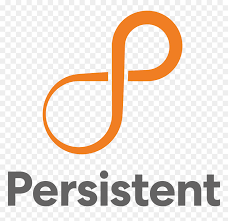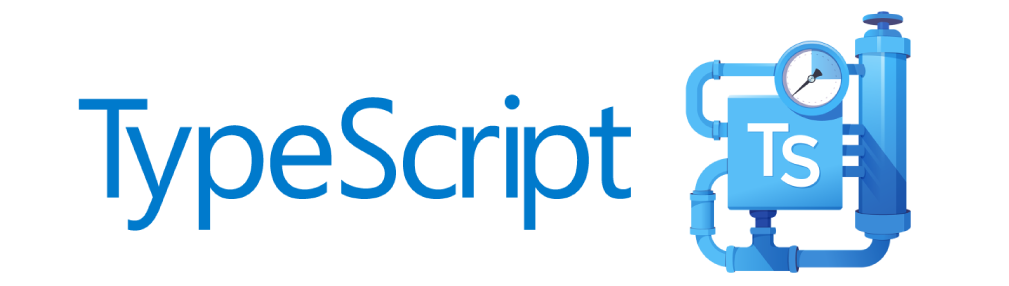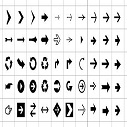# Incedo Interview questions Experienced Python Djnago

Round 1: Programming Test

1. Find four elements a, b, c and d in an array such that a+b = c+d
```Input:   {3, 4, 7, 1, 2, 9, 8}
Output:  (3, 8) and (4, 7)
Explanation: 3+8 = 4+7

Input:  {65, 30, 7, 90, 1, 9, 8};
Output:  No pairs found
```

#### Solution

```def getpairs(elements):
d=dict()
l=len(elements)
for i in range(l-1):
for j in range(i+1,l):
s=elements[i]+elements[j]
if s not in d:
d[s]=(elements[i],elements[j])
else:
print("pairs are %s and (%d,%d)"%(str(d[s]),elements[i],elements[j]))
getpairs([3, 4, 7, 1, 2, 9, 8])```
2. Given a number N, find the largest prime factor of that number.
```Input: 90
Output: 5
Input: 84
Output: 7
```

#### Solution

```def findMaxPrime(n):
while n % 2 == 0:
mp = 2
n = n / 2
for i in range(3, int(n), 2):
while n % i == 0:
mp = i
n = n / i
if n > 2:
mp = n
return int(mp)

print(findMaxPrime(84))
```
3. Given two given numbers a and b where 1<=a<=b, find the number of perfect squares between a and b (a and b inclusive).
```Input: a = 9, b = 25
Output: 3
The three squares in given range are 9,
16 and 25
```

#### Solution

```import math

def getNumberOfSqaures(a, b):
return (math.floor(math.sqrt(b)) - math.ceil(math.sqrt(a)) + 1)

print("getNumberOfSqaures :", getNumberOfSqaures(9, 25))

```
4. Given a m x n matrix, if an element is 0, set its entire row and column to 0.

#### Solution

```
def makeZero(grid):
row = len(grid)
col = len(grid)
r, c = list(), list()
for i in range(row):
for j in range(col):
if grid[i][j] == 0:
r.append(i)
c.append(j)
for i in range(row):
for j in range(col):
if i in r or j in c:
grid[i][j] = 0

grid = [
[1, 1, 1],
[1, 0, 1],
[1, 1, 1]
]
print("input -> ", grid)
makeZero(grid)
print("Output -> ", grid)

```

Round 2: Video call interview

1. Let's have a string input = "hello world 55 test 77" then print the sum of digits of numbers in pair. output = (10,14). Don't use regular expression.

Solution
```def getSum(string):
"""Without using regular expression"""
k = 0
found = False
s = 0
d = list()
for i in string + " ":
if i.strip() in [str(j) for j in range(10)]:
s = s + int(i)
found = True
k = k + 1
else:
found = False
d.append(s)
s = 0
k = 0
print(tuple(d))

getSum("hello world 55 test 77")
```
2. How to give time out error when a process takes more than the expected time ? Raise the error and kill the process

#### Solution

```import time
import multiprocessing

def raise_time_out():
p = multiprocessing.Process(target=testtime, args=)
p.start()
p.join(5)
if p.is_alive():
print('Process taking more than 5 seconds..Lets kill it')
p.terminate()
p.join()

if __name__ == "__main__":
start = time.perf_counter()
raise_time_out()
end = time.perf_counter()
print(f'Processing finished in {end - start} seconds')
```
3. What are the python libraries you have used ? Answer - math, requests, re, random, bs4, numpy, pandas etc..
4. What are the technologies you are using in your current project ? Answer - Python, Django, Oracle Data base, GiT etc..
5. How will you design an app to get the lowest fare for a bus ticket. The design should have details about source, destination, lowest fare. Give the details and code for Model, View and Templates used in Django.
6. Parent and Base classes in python ?
7. What is yield in python ?
8. What does tail command do in UNIX ?Nupur on Sep 05, 2020 at 10:09 am

#### Post Comment

##### INTERVIEW EXPERIENCES##### Google 9+ Years experience Customer Solution Engineer##### UBS interview Questions ( PLSQL 6 Years Experience)##### LNT Infotech-Python Developer Interview Questions Experienced##### Mindfire Solutions Python developer Interview Questions Experienced##### Onit India Pvt Ltd - Python Developer Interview questions Experienced##### Incedo Interview questions Experienced Python Djnago##### Python Developer - Kapstone LLC Pune Location - Experienced Interview Questions##### NICE Interactive Solutions Interview Questions Experienced PL/SQL##### Persistent Interview Experience C++##### Attra Interview Experience (PL/SQL 7 Years Experience)##### TypeScript Programmning Language##### New HTML5 APIs##### HTML Arrow -> Range: Decimal 8592-8703. Hex 2190-21FF.##### How To Override One CSS Class With Another×